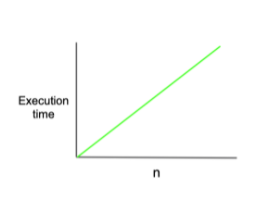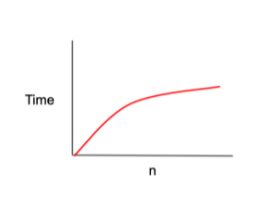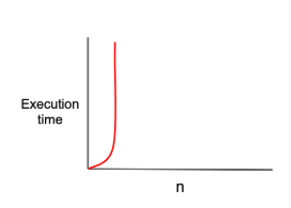# [CS] Greedy Algorithm / Implementation Day-72

cptkuk91·2022년 3월 2일
1

## CS

목록 보기
118/139Life is C(Choice) between B(Birth) and D(Death)

## What is Algorithm?

It is the best choice to solve the problem.

### Time Complexity

It is important to find the correct answer to a problem, but it is also important to solve the problem in an efficient method.

Finding an efficient method is one of the ways to solve time complexity.

### Big-O?

How to express time complexity.

• Big-O
• Big-Ω
• Big-θ

The above three notations tell us whether it is the best or the worst.

#### O(n) === 'linear complexity'

As the input increases, time also increases at the same rate.
If you input double value, Time takes twice as long.function O_n_algorithm(n) {
for (let i = 0; i < n; i++) {
// do something for 1 second
}
}

#### O(log n) === 'logarithmic complexity'

Binary Search Tree is example of logarithmic complexity. Each time to move a Node, Time to find results is cut in half.let i = n;
while (parseInt(i) > 0) {
i = i / 2;
}

ex) O(log k n) or O(log n)

for (let i = 0; i < n; i++) {
i *= k;
}

As the input increases, the time increases as a power of n.

• n^2, n^3, n^5 is all same cases
function O_quadratic_algorithm(n) {
for (let i = 0; i < n; i++) {
for (let j = 0; j < n; j++) {
// do something for 1 second
}
}
}

#### O(2^n) === 'exponential complexity'

This is the case with the slowest time complexity. need to find another efficient way.function fibonacci(n) {
if (n <= 1) {
return 1;
}
return fibonacci(n - 1) + fibonacci(n - 2);
}

O(log n) is not always faster than O(n)
As n gets bigger, O(log n) gets faster. If n is 1, the speed is the same.

## Greedy Algorithm

• Selection Procedure: Choose the best solution in the current state
• Feasibility Check: Check the selected method satisfies the condition of the problem
• Solution Check: Check the problem is solved, and if it's not, return to the Selection Procedure.

Greedy Algorithm is problem-solving method that always finds the optimal solution. However, it does not always guarantee optimal results.

## Implementation

Must know exactly the grammar of the programming language you have chosen, and the goal is to quickly write code that meets all the conditions of the problem without mistakes.

Coding requires concentration and persistence.

### brute force

All problems can be solved with brute force. It's a simple method, but you can always find the answer. But it's not efficient method.

### simulation

Thinking about how to solve a problem can be easy, but writing code is hard.

If you omit even one condition, it's not easy to find the correct answer.메일은 매일 확인하고 있습니다. 궁금하신 부분이나 틀린 부분에 대한 지적사항이 있으시다면 언제든 편하게 연락 부탁드려요 :)